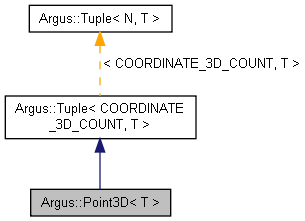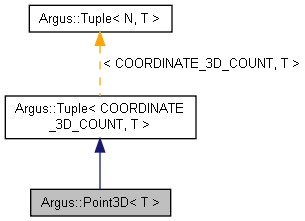# Jetson Linux API Reference

##### 32.7.1 Release
Argus::Point3D< T > Class Template Reference

## Detailed Description

### template<typename T> class Argus::Point3D< T >

Point3D template class.

This is a Tuple specialization containing 3 elements corresponding to the x, y and z coordinates of a 3D point. Values can be accessed using the named methods or subscript indexing using the Argus::Coordinate enum.

Definition at line 674 of file Types.h.

Inheritance diagram for Argus::Point3D< T >:[legend]
Collaboration diagram for Argus::Point3D< T >:[legend]

## Public Member Functions

Point3D ()

Point3D (const Tuple< COORDINATE_3D_COUNT, T > &other)

Point3D (T init)

Point3D (T _x, T _y, T _z)

T & x ()

const T & x () const

T & y ()

const T & y () const

T & z ()

const T & z () const

bool operator== (const Tuple< N, T > &rhs) const
Returns true when every element in the two tuples are identical. More...

bool operator!= (const Tuple< N, T > &rhs) const
Returns true if there are any differences between the two tuples. More...

Tuple< N, T > & operator+= (const Tuple< N, T > &rhs)
Adds every element of another tuple to the elements of this tuple. More...

Tuple< N, T > & operator-= (const Tuple< N, T > &rhs)
Subtracts every element of another tuple from the elements of this tuple. More...

Tuple< N, T > & operator*= (const T &rhs)
Multiplies every element in the tuple by a single value. More...

Tuple< N, T > & operator/= (const T &rhs)
Divides every element in the tuple by a single value. More...

const Tuple< N, T > operator+ (const Tuple< N, T > &rhs) const
Returns the result of adding another tuple to this tuple. More...

const Tuple< N, T > operator- (const Tuple< N, T > &rhs) const
Returns the result of subtracting another tuple from this tuple. More...

const Tuple< N, T > operator* (const T &rhs) const
Returns the result of multiplying this tuple by a single value. More...

const Tuple< N, T > operator/ (const T &rhs) const
Returns the result of dividing this tuple by a single value. More...

T & operator[] (unsigned int i)

const T & operator[] (unsigned int i) const

## Static Public Member Functions

static unsigned int tupleSize ()
Returns the number of elements in the tuple. More...

m_data [N]

## ◆ Point3D() [1/4]

template<typename T >
 Argus::Point3D< T >::Point3D ( )
inline

Definition at line 677 of file Types.h.

## ◆ Point3D() [2/4]

template<typename T >
 Argus::Point3D< T >::Point3D ( const Tuple< COORDINATE_3D_COUNT, T > & other )
inline

Definition at line 678 of file Types.h.

## ◆ Point3D() [3/4]

template<typename T >
 Argus::Point3D< T >::Point3D ( T init )
inline

Definition at line 680 of file Types.h.

## ◆ Point3D() [4/4]

template<typename T >
 Argus::Point3D< T >::Point3D ( T _x, T _y, T _z )
inline

Definition at line 685 of file Types.h.

## ◆ operator!=()

 bool Argus::Tuple< N, T >::operator!= ( const Tuple< N, T > & rhs ) const
inlineinherited

Returns true if there are any differences between the two tuples.

Definition at line 500 of file Types.h.

## ◆ operator*()

 const Tuple Argus::Tuple< N, T >::operator* ( const T & rhs ) const
inlineinherited

Returns the result of multiplying this tuple by a single value.

Definition at line 550 of file Types.h.

## ◆ operator*=()

 Tuple& Argus::Tuple< N, T >::operator*= ( const T & rhs )
inlineinherited

Multiplies every element in the tuple by a single value.

Definition at line 522 of file Types.h.

## ◆ operator+()

 const Tuple Argus::Tuple< N, T >::operator+ ( const Tuple< N, T > & rhs ) const
inlineinherited

Returns the result of adding another tuple to this tuple.

Definition at line 538 of file Types.h.

## ◆ operator+=()

 Tuple& Argus::Tuple< N, T >::operator+= ( const Tuple< N, T > & rhs )
inlineinherited

Adds every element of another tuple to the elements of this tuple.

Definition at line 506 of file Types.h.

## ◆ operator-()

 const Tuple Argus::Tuple< N, T >::operator- ( const Tuple< N, T > & rhs ) const
inlineinherited

Returns the result of subtracting another tuple from this tuple.

Definition at line 544 of file Types.h.

## ◆ operator-=()

 Tuple& Argus::Tuple< N, T >::operator-= ( const Tuple< N, T > & rhs )
inlineinherited

Subtracts every element of another tuple from the elements of this tuple.

Definition at line 514 of file Types.h.

## ◆ operator/()

 const Tuple Argus::Tuple< N, T >::operator/ ( const T & rhs ) const
inlineinherited

Returns the result of dividing this tuple by a single value.

Definition at line 556 of file Types.h.

## ◆ operator/=()

 Tuple& Argus::Tuple< N, T >::operator/= ( const T & rhs )
inlineinherited

Divides every element in the tuple by a single value.

Definition at line 530 of file Types.h.

## ◆ operator==()

 bool Argus::Tuple< N, T >::operator== ( const Tuple< N, T > & rhs ) const
inlineinherited

Returns true when every element in the two tuples are identical.

Definition at line 494 of file Types.h.

## ◆ operator[]() [1/2]

 T& Argus::Tuple< N, T >::operator[] ( unsigned int i )
inlineinherited

Definition at line 561 of file Types.h.

## ◆ operator[]() [2/2]

 const T& Argus::Tuple< N, T >::operator[] ( unsigned int i ) const
inlineinherited

Definition at line 562 of file Types.h.

## ◆ tupleSize()

 static unsigned int Argus::Tuple< N, T >::tupleSize
inlinestaticinherited

Returns the number of elements in the tuple.

Definition at line 565 of file Types.h.

## ◆ x() [1/2]

template<typename T >
 T& Argus::Point3D< T >::x ( )
inline

Definition at line 692 of file Types.h.

References Argus::COORDINATE_X.

Referenced by Argus::Point3D< T >::Point3D().

## ◆ x() [2/2]

template<typename T >
 const T& Argus::Point3D< T >::x ( ) const
inline

Definition at line 693 of file Types.h.

References Argus::COORDINATE_X.

## ◆ y() [1/2]

template<typename T >
 T& Argus::Point3D< T >::y ( )
inline

Definition at line 694 of file Types.h.

References Argus::COORDINATE_Y.

Referenced by Argus::Point3D< T >::Point3D().

## ◆ y() [2/2]

template<typename T >
 const T& Argus::Point3D< T >::y ( ) const
inline

Definition at line 695 of file Types.h.

References Argus::COORDINATE_Y.

## ◆ z() [1/2]

template<typename T >
 T& Argus::Point3D< T >::z ( )
inline

Definition at line 696 of file Types.h.

References Argus::COORDINATE_Z.

Referenced by Argus::Point3D< T >::Point3D().

## ◆ z() [2/2]

template<typename T >
 const T& Argus::Point3D< T >::z ( ) const
inline

Definition at line 697 of file Types.h.

References Argus::COORDINATE_Z.

## ◆ m_data

 T Argus::Tuple< N, T >::m_data[N]
protectedinherited

Definition at line 568 of file Types.h.

The documentation for this class was generated from the following file: